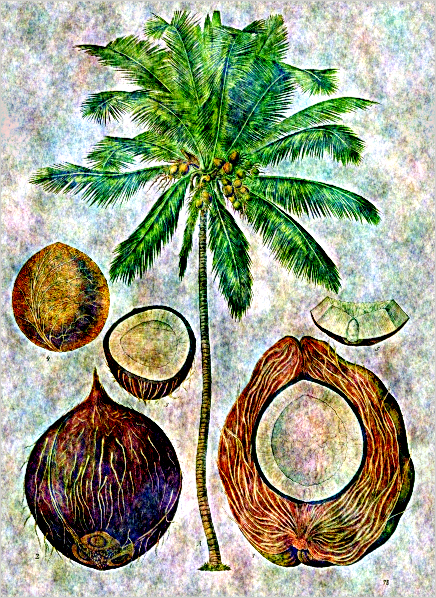# Coco notesThese notes are mostly for personal use, so that i do not forget the things that took some time to learn. There is no pretense of novelty anywhere.

The source files below are often literate programs: a single file is used to run the experiments and to render the result into html and pdf. All the notes are thus built by a simple makefile. For image-heavy documents, the html output is recommended because sets of related images are collected into galleries (the pdf file will have many pages with one image on each).

## 1. Image processing

Image processing with graphs, html, pdf, tex
We propose octave implementations of graph-based mathematical morphology and linear PDE for image processing.
The canonical noise of an image, html, pdf, tex
A cute trick for adding noise without recourse to pseudo-random numbers.
Linear dithering, html, pdf, tex
We explore the space of binarization techniques that lie between thresholding and dithering.
Gallery of single-layer textures, html, pdf, tex
We show how single layer CNN can hallucinate snowflakes and dandelions.
Colors of image noise, html, pdf, tex
We show gaussian noise which is not necessarily white.
Imscript tutorial, html,
A tutorial for some of the programs from imscript.

## 2. Math

One-dimensional clustering with one cluster html, pdf, tex
A comprehensive study of the problem of combining several numbers into one.
Generalizations of Fourier analyisis, html, pdf, tex
We explain some common generalizations of Fourier analysis: sampling theory, distribution theory, pontryagin duality and spectral geometry. The part on spectral theory is shared with the article about PDE on manifolds (needs some editing yet)
The standard PDE on a manifold html, pdf, tex
We state and solve the standard linear PDE on a compact Riemannian manifold.
Algebraic Graph Calculus html, pdf, tex
We do graph theory as if it was vector calculus.
The Cauchy basis html, pdf, tex
We introduce a sequence of functions that satisfy Dirichlet and Neumann boundary conditions at the same time.

## 3. Programming

FTR, html
A portable and ported API for simple graphical interactive programs.
NIPL, txt
NIPL is Not an Image Processing Libray
Makefiles for mathematicians, html
Why and how to enjoy Makefiles
How to write sane C++, wiki
A very short but very strict set of conventions for programming in C++
How to write sane C, wiki
An elaborate set of conventions for programming in C
How to write sane shell scripts, txt
An simple set of conventions for shell scripting.
How to write sane python, txt
Very un-pythonic conventions for programming in python
Zero boilerplate, html. pdf.